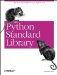# The dbhash Module

(Optional) The dbhash module provides a dbm-compatible interface to the bsddb database handler. Example 10-5 uses this module.

Example 10-5. Using the dbhash Module

```File: dbhash-example-1.py

import dbhash

db = dbhash.open("dbhash", "c")
db["one"] = "the foot"
db["two"] = "the shoulder"
db["three"] = "the other foot"
db["four"] = "the bridge of the nose"
db["five"] = "the naughty bits"
db["six"] = "just above the elbow"
db["seven"] = "two inches to the right of a very naughty bit indeed"
db["eight"] = "the kneecap"
db.close()

db = dbhash.open("dbhash", "r")
for key in db.keys():
print repr(key), repr(db[key])```Python Standard Library (Nutshell Handbooks) with
ISBN: 0596000960
EAN: 2147483647
Year: 2000
Pages: 252
Authors: Fredrik Lundh